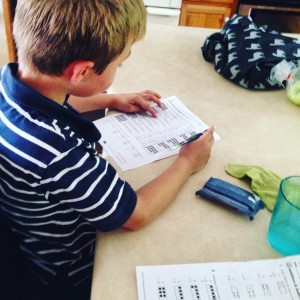# The Tutoring Center, Franklin TN

01/12/2018

## How to Improve Your Child's Mental Math SkillsBeing able to recall simple math facts quickly is a great asset for students of all ages. To help ensure your child is able to solve simple math equations in their head, have them practice their mental math skills at home. Use these tips to get them on the right track.

## Memorize Easy Math

There are certain easy math facts that students can benefit from memorizing. For example, memorizing multiplication tables can make it easier for students to solve longer math problems more efficiently. Other information they may benefit from memorizing includes simple squares, square roots, and 1/n's.

## Break Down Problems

In order to avoid pulling out a calculator, teach your child to use shortcuts that can make solving a simple equation easier. For example, if your child is trying to figure out the answer to 5x8 make it easier by multiplying the 5 by 2 so that the equation is then 10x8. Multiplying by 10 is much easier for most students, making the equation much less intimidating. Once your child has the answer, 80, have them divide it by 2. Dividing by 2 is also quite simple for most students because its a low number. Your child should then be left with the answer, 40.

## Use Speed Drills

Most students are able to memorize their multiplication tables thanks to speed drills used in school. These are basically work sheets with a number of multiplication problems that must be completed in a minute. You can use worksheets or even websites that include a timer to get your child to practice thinking fast.

## Math Tutoring in Franklin TN

No matter what type of math your child is struggling with, tutoring in Franklin TN can help them become a math expert. Contact The Tutoring Center, Franklin TN at (615)721-8844 to get your child enrolled in a great math tutoring program.

### FREE CONSULTATION

Schedule your Free Diagnostic Assessment Today!